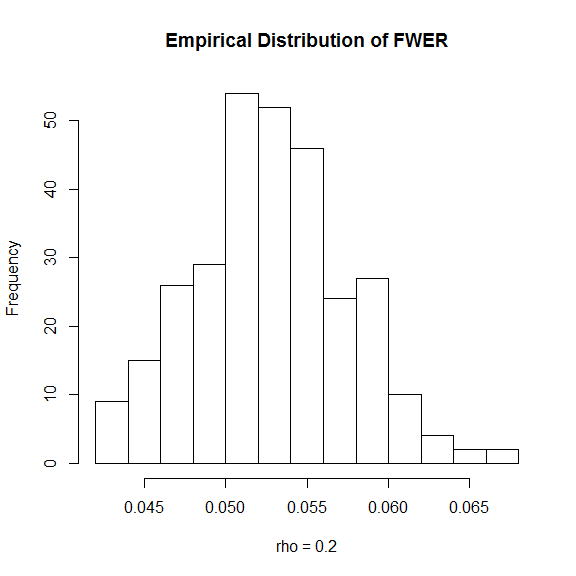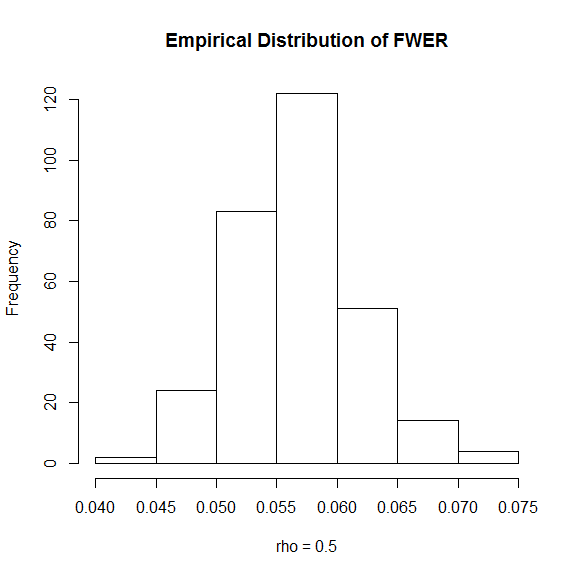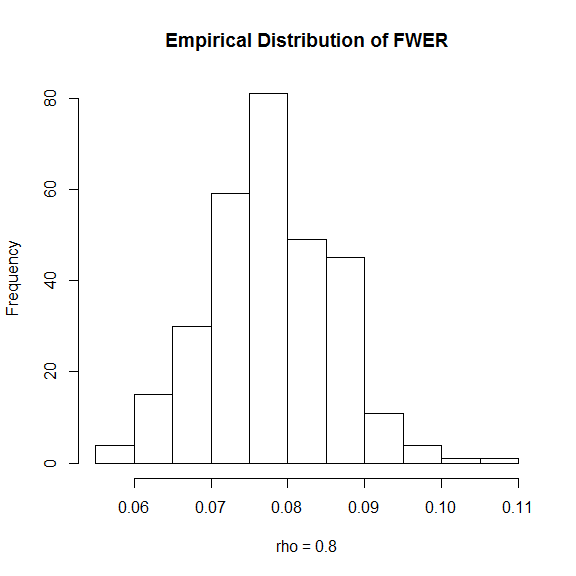# Using the FWER controlling z-test procedure of Stange, Bodnar, Dickhaus (2015)

#### 2018-04-05

This vignette demonstrates the usage of the FWER controlling z-test described in J. Stange, T. Bodnar and T. Dickhaus (2015). The paper is available at https://doi.org/10.1007/s10182-014-0241-5. All following references to equations and theorems refer to this paper.

## Performing FWER controlling z-tests using fwer.ztest

Let $$X_1,\cdots,X_n$$ denote an i.i.d. sample with values in $$\mathbb{R}^m$$. Furthermore let $$\mu_j=\mathbb{E}\left[X_{1,j}\right]$$ ($$j=1,\cdots,m$$) be the component-wise expectations. Then the multiple (two-sided) z-test simultaneously tests the hypotheses $$H_{0,j}: \mu_j = \mu_j^*$$ versus the corresponding alternatives $$H_{1,j}: \mu_j\not=\mu_j^*$$.

For values drawn from Model 1a) the test can be performed using fwer.ztest as follows:

set.seed(0)
Sigma_GaussianAR1<-function(rho,m) {
exponents<-abs(outer(1:m,1:m,"-")) #exponents for AR(1) matrix
Sigma<-rho^exponents               #computes the AR(1) matrix
return(Sigma)
}
n <- 100
m <- 10
m0 <- 5
mu <- c(rep(0,m0),runif(m-m0))
sample <- mvtnorm::rmvnorm(n,mu,Sigma_GaussianAR1(0.1,m))
res <- fwer.ztest(sample,rep(0,m))
res$test$Empirical
#>   FALSE FALSE FALSE FALSE FALSE  TRUE FALSE  TRUE  TRUE  TRUE

Thus only the hypothesis $$H_{0,7}$$ is wrongly not rejected.

## Figures 2 and 3: Empirical power and FWER for model 1a) (Gaussian with AR(1) covariance matrix)

The power and FWER of the procedure under model 1a) are estimated using a Monte carlo simulation. To this end samples are repeatedly drawn from a normal distribution

sig <- Sigma_GaussianAR1(rho,m)
samplefun <- function(mu) mvtnorm::rmvnorm(n,mu,sig)

and then the test is applied:

testfun <- function(X) fwer.ztest(X,rep(0,m))

To see the full code for generating the figures execute the following code:

v <- vignette('fwer-ztest',package = 'MHTcop')
file.edit(paste(v$Dir,'doc',v$R,sep='/'))

### Figure 2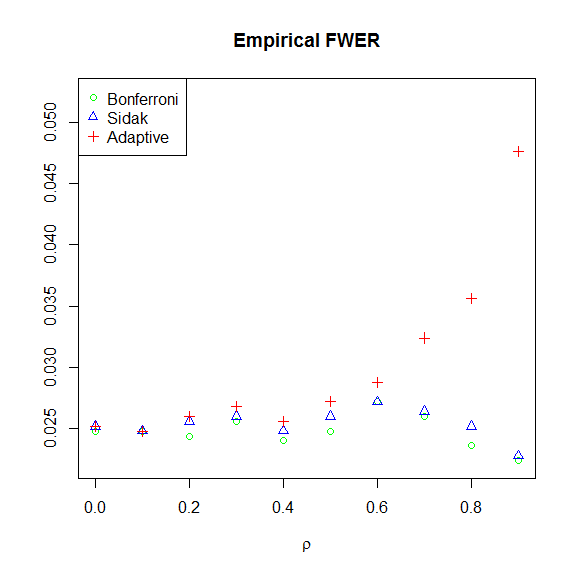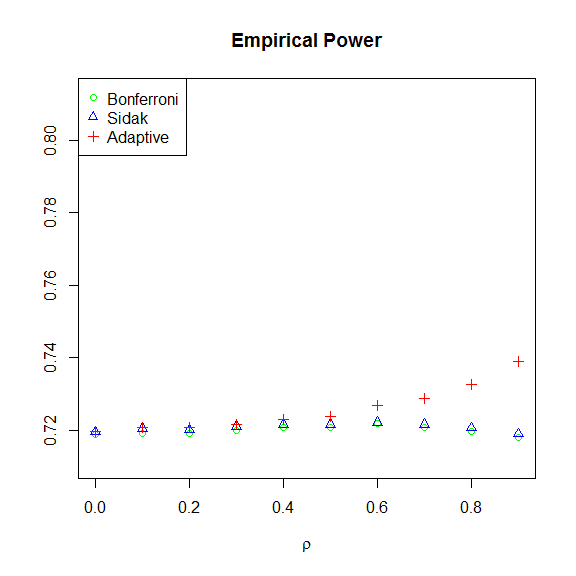### Figure 3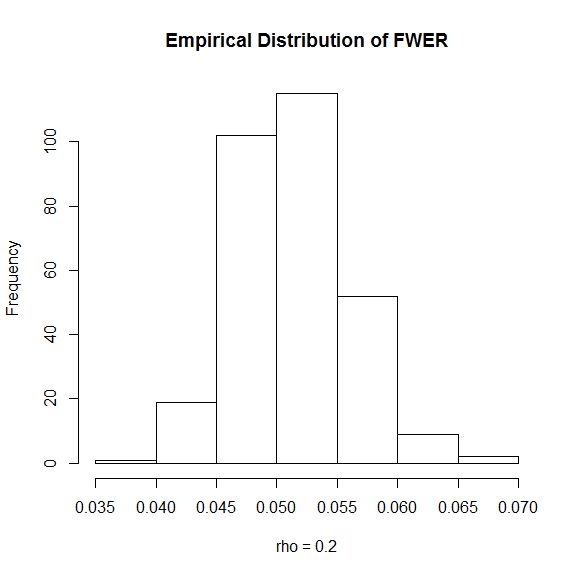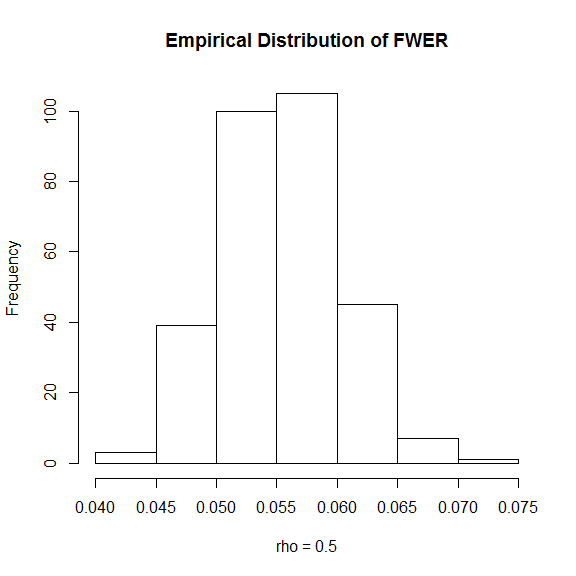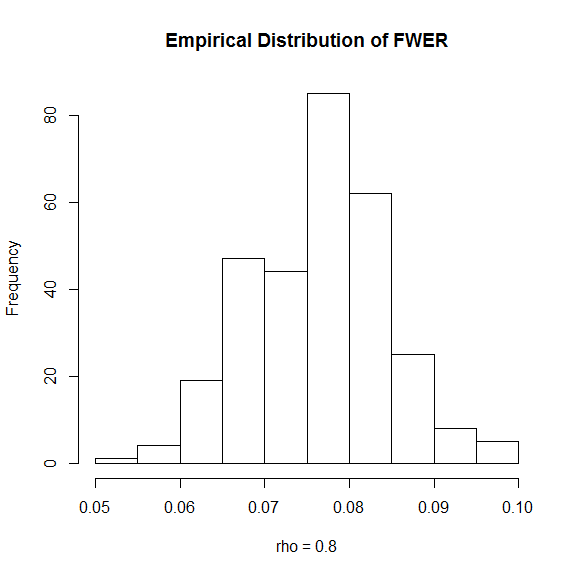## Figures 4 and 5: Empirical power and FWER for model 1b) (t-distribution with 9 degrees of freedom and AR(1) covariance matrix)

The power and FWER of the procedure under model 1b) are estimated using a Monte carlo simulation. To this end samples are repeatedly drawn from a t-distribution

Sigma_T_AR1<-function(m=2,rho=0,nu=3) {
exponents<-abs(outer(1:m,1:m,"-"))  #exponents for AR(1) matrix
Sigma<-(nu-2)/nu*rho^exponents      #computes the AR(1) matrix
return(Sigma)
}
samplefun <- function(mu) {
return(t(mu+t(mvtnorm::rmvt(n,sigma=sig,df=nu))))
}

and then the test is applied:

sig <- Sigma_T_AR1(m,rho,nu)
testfun <- function(X) fwer.ztest(X,rep(0,m))

### Figure 4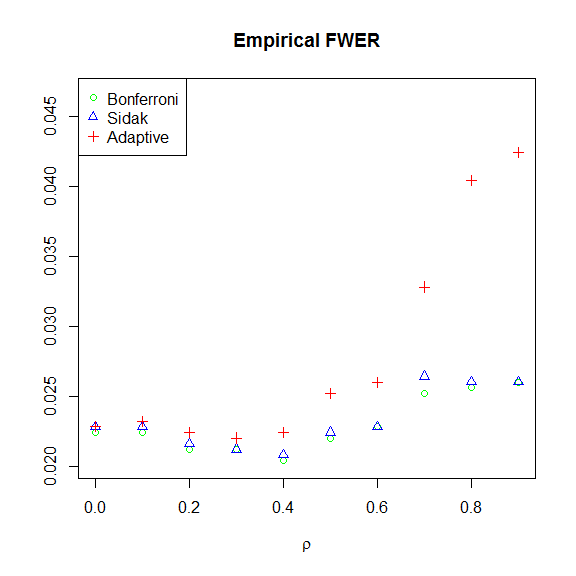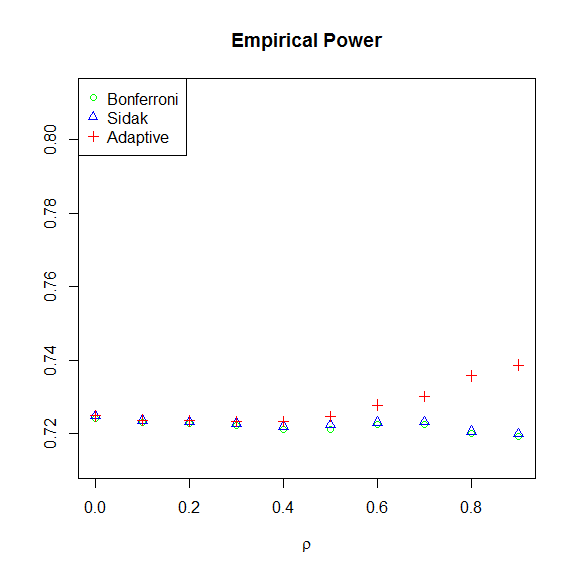### Figure 5